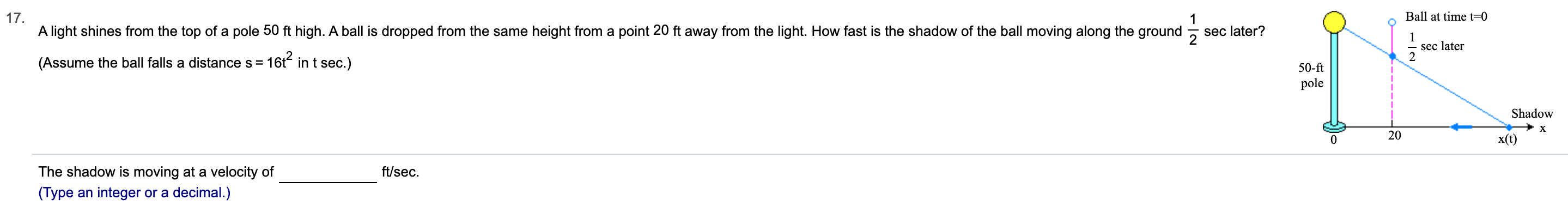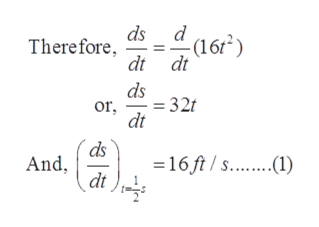# 17A light shines from the top of a pole 50 ft high. A ball is dropped from the same height from a point 20 ft away from the light. How fast is the shadow of the ball moving along the groundBall at time t-01sec later?2sec later16t2 in t sec.)(Assume the ball falls a distance s50-ftpoleShadowX20x(t)The shadow is moving at a velocity offt/sec(Type an integer or a decimal.)

Question
3 views

Can you help me step by step?help_outlineImage Transcriptionclose17 A light shines from the top of a pole 50 ft high. A ball is dropped from the same height from a point 20 ft away from the light. How fast is the shadow of the ball moving along the ground Ball at time t-0 1 sec later? 2 sec later 16t2 in t sec.) (Assume the ball falls a distance s 50-ft pole Shadow X 20 x(t) The shadow is moving at a velocity of ft/sec (Type an integer or a decimal.) fullscreen
check_circle

Step 1

Let, the horizontal distance between shadow and pole is x. And s= 16 t2 represents the vertical distance when the ball falls in time (t) seconds.help_outlineImage Transcriptioncloseds - (16r2) dt dt Therefore ds or, dt = 32 32t ds And 16 ft/s... dt fullscreen
Step 2

The distance travelled by ball after 0.5 seconds is s (say).

Step 3

Using properties of sim...

### Want to see the full answer?

See Solution

#### Want to see this answer and more?

Solutions are written by subject experts who are available 24/7. Questions are typically answered within 1 hour.*

See Solution
*Response times may vary by subject and question.
Tagged in

### Calculus